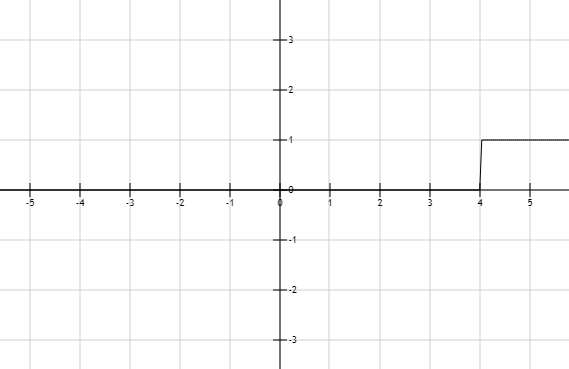# The solution of given linear inequality equation and plot a graph for it.### Precalculus: Mathematics for Calcu...

6th Edition
Stewart + 5 others
Publisher: Cengage Learning
ISBN: 9780840068071### Precalculus: Mathematics for Calcu...

6th Edition
Stewart + 5 others
Publisher: Cengage Learning
ISBN: 9780840068071

#### Solutions

Chapter 1.7, Problem 13E
To determine

## The solution of given linear inequality equation and plot a graph for it.

Expert Solution

x>4

### Explanation of Solution

Given:

Linear inequality equation

2x5>3

Calculation,

Linear inequality equation,

2x5>32x>3+52x>8x>82x>4

Now, graph of 2x5>3Conclusion:

Hence, the values of x is greater than equal to the 4.

### Have a homework question?

Subscribe to bartleby learn! Ask subject matter experts 30 homework questions each month. Plus, you’ll have access to millions of step-by-step textbook answers!